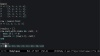In Progress
Unit 1, Lesson 1
In Progress

# eachVideo transcript & code

Recently I started playing around with maze generation algorithms. One way to view a maze from the point of view of a computer is as an undirected graph, where the maze cells are the nodes and the walls are edges between nodes.

To play around with this idea, let's make a 5x5 board full of cells. We'll use a `Cell` class which has just one property: a cell name.

``````class Cell
include Comparable

def initialize(name)
@name = name
end

def inspect
@name
end

def <=>(other)
name <=> other.name
end

def hash
[self.class, name].hash
end

alias_method :eql?, :==
end
``````

To represent a grid of cells, we can use a nested map to generate 5 rows of 5 cells each. For easy identification, we'll give each cell a single-letter name, from "a" through "y".

``````require "./cell"
name = "a"
BOARD = 5.times.map do |y|
5.times.map do |x|
cell = Cell.new(name)
name = name.succ
cell
end
end
``````

Let's visualize this board.

``````board
# => [[a, b, c, d, e],
#     [f, g, h, i, j],
#     [k, l, m, n, o],
#     [p, q, r, s, t],
#     [u, v, w, x, y]]
``````

Now, in order to represent this grid as a graph, we need to generate graph edges for each wall. So we need an edge for the wall between "a" and "b", the wall between "b" and "c", and so on. There is no edge between "e" and "f", though, because they straddle different rows.

Let's start off by just doing this operation for the top row.

The naive way to do this might be to use a loop. We set up an empty array for the results. Then for each item in the list, we add that item plus the item before it into a two-element array. We exclude the first iteration, because at that point there is no preceding item.

``````require "./board"

row = BOARD.first
# => [a, b, c, d, e]

results = []
row.each_with_index do |cell, i|
next if i == 0
results << [row[i-1], cell]
end

results
# => [[a, b], [b, c], [c, d], [d, e]]
``````

This works. We can see the pairs of a and b, b and c, and so on.

But it's a clunky way to go about it. You might be wondering if somewhere in the `Enumerable` bag of tricks there's a tool to make this easier. And as usual with Ruby `Enumerables`, if you can wonder about it, it probably exists.

Instead of writing a loop, we can simply send `#each_cons`, which is short for "each consecutive". We give it a number, which is the number of consecutive items to return at a time. This is a block-taking method, so we can output each chuck as it is generated:

``````require "./board"

row = BOARD.first
# => [a, b, c, d, e]

row.each_cons(2) do |cons|
p cons
end

# >> [a, b]
# >> [b, c]
# >> [c, d]
# >> [d, e]
``````

But rather than passing a block, we can also use the `Enumerator` it returns to generate an array.

``````require "./board"

row = BOARD.first
# => [a, b, c, d, e]

row.each_cons(2).to_a
# => [[a, b], [b, c], [c, d], [d, e]]
``````

When we look at results, we can think of them as being generated by a kind of "sliding window". We have a window that is two elements wide, and we are sliding it one element at a time across the original array, and saving a snapshot each time.

That gives us our first row. Now let's expand it out to the whole grid. We can use `#flat_map`, which we met in episode #285 to map through each row without generating extra nesting. For each row, we return the array-ified results of `#each_cons`. Then we convert the `#flat_map` enumerator into an array, and take a look at the results.

``````require "./board"

walls = BOARD.flat_map{|row| row.each_cons(2).to_a}.to_a
# => [[a, b],
#     [b, c],
#     [c, d],
#     [d, e],
#     [f, g],
#     [g, h],
#     [h, i],
#     [i, j],
#     [k, l],
#     [l, m],
#     [m, n],
#     [n, o],
#     [p, q],
#     [q, r],
#     [r, s],
#     [s, t],
#     [u, v],
#     [v, w],
#     [w, x],
#     [x, y]]
``````

Going down this list, we can see that we have entries for a to b; b to c; c to d; and d to e; but there is no e to f because f is on the next row. This is exactly the list of graph edges we wanted.

Of course, these are only the horizontal or "longitudinal" walls. We have yet to generate the latitudinal edges. But we'll need a new trick for that, and it can wait until the next episode. Happy hacking!